Anzeige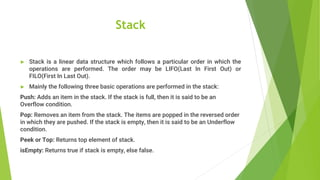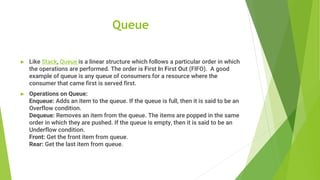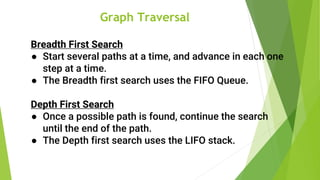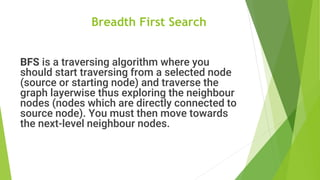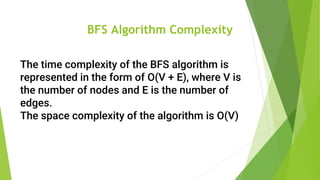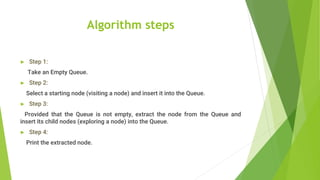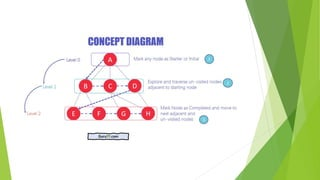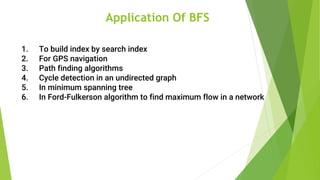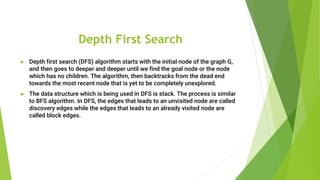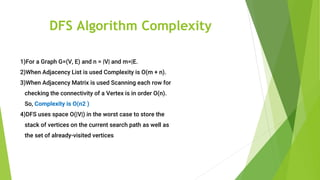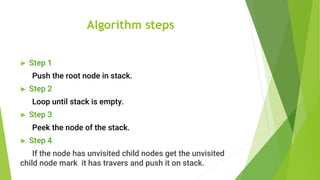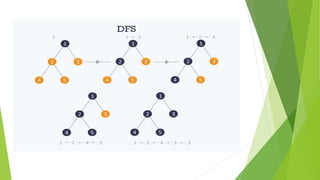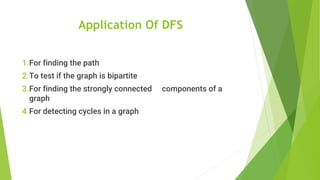1 von 15
Anzeige

### Bfs and dfs

1. Breadth First Search & Depth First Search Ritesh Thakur Vishal Thoke Utsav Patel Sushant Vaidkar Vedant Valsangkar
2. Stack ► Stack is a linear data structure which follows a particular order in which the operations are performed. The order may be LIFO(Last In First Out) or FILO(First In Last Out). ► Mainly the following three basic operations are performed in the stack: Push: Adds an item in the stack. If the stack is full, then it is said to be an Overflow condition. Pop: Removes an item from the stack. The items are popped in the reversed order in which they are pushed. If the stack is empty, then it is said to be an Underflow condition. Peek or Top: Returns top element of stack. isEmpty: Returns true if stack is empty, else false.
3. Queue ► Like Stack, Queue is a linear structure which follows a particular order in which the operations are performed. The order is First In First Out (FIFO). A good example of queue is any queue of consumers for a resource where the consumer that came first is served first. ► Operations on Queue: Enqueue: Adds an item to the queue. If the queue is full, then it is said to be an Overflow condition. Dequeue: Removes an item from the queue. The items are popped in the same order in which they are pushed. If the queue is empty, then it is said to be an Underflow condition. Front: Get the front item from queue. Rear: Get the last item from queue.
4. Graph Traversal Breadth First Search ● Start several paths at a time, and advance in each one step at a time. ● The Breadth first search uses the FIFO Queue. Depth First Search ● Once a possible path is found, continue the search until the end of the path. ● The Depth first search uses the LIFO stack.
5. Breadth First Search BFS is a traversing algorithm where you should start traversing from a selected node (source or starting node) and traverse the graph layerwise thus exploring the neighbour nodes (nodes which are directly connected to source node). You must then move towards the next-level neighbour nodes.
6. BFS Algorithm Complexity The time complexity of the BFS algorithm is represented in the form of O(V + E), where V is the number of nodes and E is the number of edges. The space complexity of the algorithm is O(V)
7. Algorithm steps ► Step 1: Take an Empty Queue. ► Step 2: Select a starting node (visiting a node) and insert it into the Queue. ► Step 3: Provided that the Queue is not empty, extract the node from the Queue and insert its child nodes (exploring a node) into the Queue. ► Step 4: Print the extracted node.
8. Application Of BFS 1. To build index by search index 2. For GPS navigation 3. Path finding algorithms 4. Cycle detection in an undirected graph 5. In minimum spanning tree 6. In Ford-Fulkerson algorithm to find maximum flow in a network
9. Depth First Search ► Depth first search (DFS) algorithm starts with the initial node of the graph G, and then goes to deeper and deeper until we find the goal node or the node which has no children. The algorithm, then backtracks from the dead end towards the most recent node that is yet to be completely unexplored. ► The data structure which is being used in DFS is stack. The process is similar to BFS algorithm. In DFS, the edges that leads to an unvisited node are called discovery edges while the edges that leads to an already visited node are called block edges.
10. DFS Algorithm Complexity 1)For a Graph G=(V, E) and n = |V| and m=|E. 2)When Adjacency List is used Complexity is O(m + n). 3)When Adjacency Matrix is used Scanning each row for checking the connectivity of a Vertex is in order O(n). So, Complexity is O(n2 ) 4)DFS uses space O(|V|) in the worst case to store the stack of vertices on the current search path as well as the set of already-visited vertices
11. Algorithm steps ► Step 1 Push the root node in stack. ► Step 2 Loop until stack is empty. ► Step 3 Peek the node of the stack. ► Step 4 If the node has unvisited child nodes get the unvisited child node mark it has travers and push it on stack.
12. Application Of DFS 1.For finding the path 2.To test if the graph is bipartite 3.For finding the strongly connected components of a graph 4.For detecting cycles in a graph
Anzeige